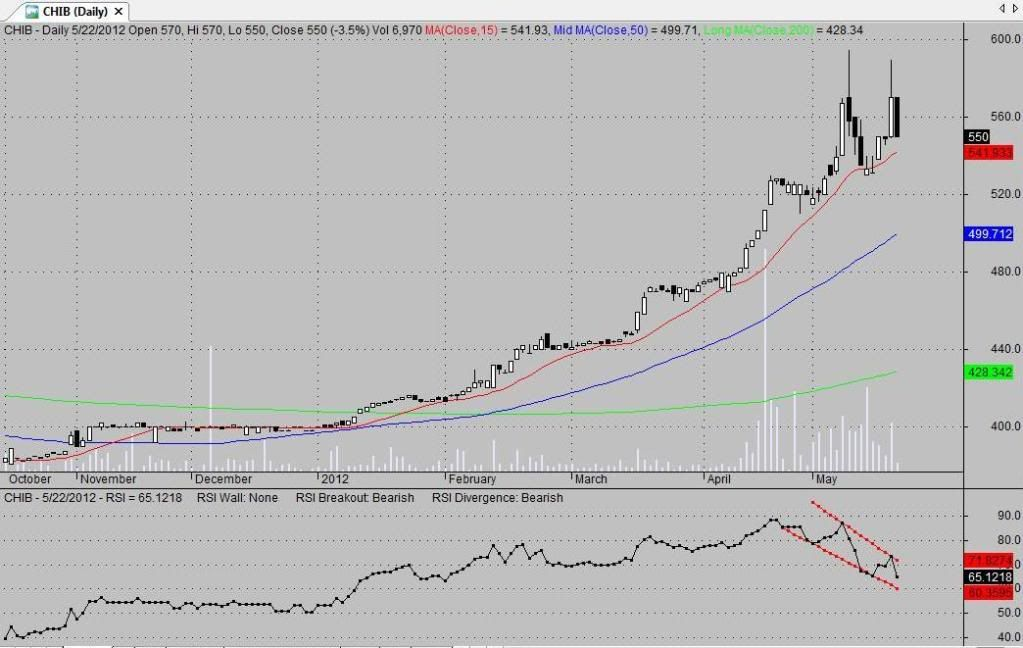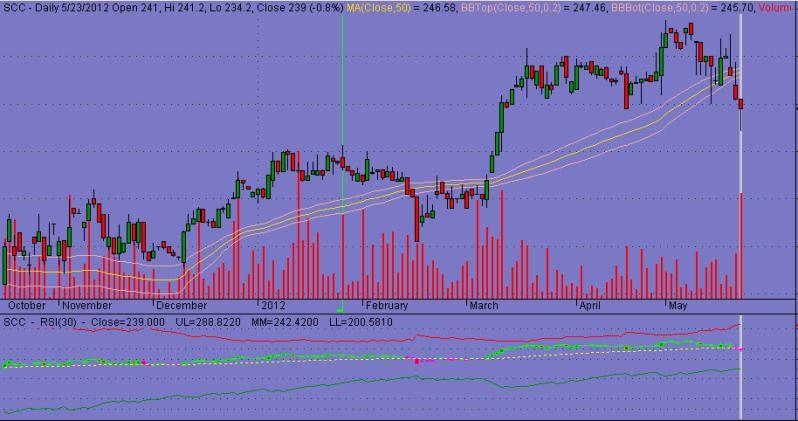AMIBROKER AFL CODEippfxSMP ElitePosts: 1,181 Threads: 7 Joined: Aug 16 2011 Reputation: 33 05-22-2012, 09:33 AM For those interested, here's an exploration for the RSI wall, breakout/penetration and divergence. The divergence rules were adapted from Metastock Expert Advisor. // RSI Breakout, Wall, Divergence Exploration period = Param("Period", 30); r = RSI(period); p2 = period / 2; // breakout bullish_break = r > Ref( HHV(r, p2),-1 ); // breakout from resistance bearish_break = r < Ref( LLV(r, p2),-1 ); // penetration of support // wall bullish_wall = r == Ref( HHV(r, p2),-1 ); // at the resistance wall bearish_wall = r == Ref( LLV(r, p2),-1 ); // at the support wall // divergence bullish_divergence1 = r >= HHV(r, p2) AND C < HHV(C, p2); bullish_divergence2 = C <= LLV(C, p2) AND r > LLV(r, p2); bearish_divergence1 = C >= HHV(C, p2) AND r < HHV(r, p2); bearish_divergence2 = r <= LLV(r, p2) AND C > LLV(C, p2); Filter = 1; walltxt = WriteIf( bullish_wall, "Bullish", WriteIf( bearish_wall, "Bearish", "None" )); breaktxt = WriteIf( bullish_break, "Bullish", WriteIf( bearish_break, "Bearish", "None" )); divtxt = WriteIf( bullish_divergence1 OR bullish_divergence2, "Bullish", WriteIf( bearish_divergence1 OR bearish_divergence2, "Bearish", "None" )); AddTextColumn(walltxt, "Wall"); AddTextColumn(breaktxt, "Breakout"); AddTextColumn(divtxt, "Divergence"); AddColumn(C, "Close", 1.4); AddColumn(LLV(C, p2), "Lowest Close", 1.4); AddColumn(HHV(C, p2), "Highest Close"); AddColumn(r, "RSI"); AddTextColumn( WriteIf( ROC(r,1) > 0, "Up", "Down" ), "Slope" ); AddColumn(LLV(r, p2), "Lowest RSI"); AddColumn(HHV(r, p2), "Highest RSI"); Cheers! "A man should look for what is, and not for what he thinks should be." - Albert EinsteinINDOModerator nga BisayaPosts: 3,104 Threads: 12 Joined: Sep 05 2011 Reputation: 117 05-23-2012, 08:07 AM Nice Boss ippfxThanks... iincorporate ko ito sa code na pwede natin makita sa chart ha para everytime na titingin tayo sa chart malalaman natin agad ang status==================================== Get a Daily Stock Guide with TheDailyHINT plus FREE Stock reports here!ippfxSMP ElitePosts: 1,181 Threads: 7 Joined: Aug 16 2011 Reputation: 33 05-23-2012, 08:32 AM i'd like to see that. pa post na lang ng chart"A man should look for what is, and not for what he thinks should be." - Albert EinsteinINDOModerator nga BisayaPosts: 3,104 Threads: 12 Joined: Sep 05 2011 Reputation: 117 05-23-2012, 12:26 PM Here's the incorporated RSI code... Quote:_SECTION_BEGIN("SMP RSI,INCORPORATION"); //code by ippfx of SMP// // RSI Breakout, Wall, Divergence Exploration period = Param("Period", 30); r = RSI(period); p2 = period / 2; // breakout bullish_break = r > Ref( HHV(r, p2),-1 ); // breakout from resistance bearish_break = r < Ref( LLV(r, p2),-1 ); // penetration of support // wall bullish_wall = r == Ref( HHV(r, p2),-1 ); // at the resistance wall bearish_wall = r == Ref( LLV(r, p2),-1 ); // at the support wall // divergence bullish_divergence1 = r >= HHV(r, p2) AND C < HHV(C, p2); bullish_divergence2 = C <= LLV(C, p2) AND r > LLV(r, p2); bearish_divergence1 = C >= HHV(C, p2) AND r < HHV(r, p2); bearish_divergence2 = r <= LLV(r, p2) AND C > LLV(C, p2); walltxt = WriteIf( bullish_wall, "Bullish", WriteIf( bearish_wall, "Bearish", "None" )); breaktxt = WriteIf( bullish_break, "Bullish", WriteIf( bearish_break, "Bearish", "None" )); divtxt = WriteIf( bullish_divergence1 OR bullish_divergence2, "Bullish", WriteIf( bearish_divergence1 OR bearish_divergence2, "Bearish", "None" )); AddTextColumn(walltxt, "Wall"); AddTextColumn(breaktxt, "Breakout"); AddTextColumn(divtxt, "Divergence"); //DOWNLOADED FROM THE OTHER FORUM with little revision// /*RSI Trendlines and Wedges*/ G=0;// set G=1, 2, 3, ... to see previous Resistance trendlines GG=0;// set GG=1, 2, 3, ... to see previous Support trendlines x = *****(1); per = 1;// sensitivity calibration s1=RSI(period);s11=RSI(period); Plot(RSI(period),"RSI ",1,8); pS = TroughBars( s1, per, 1 ) == 0; endt= LastValue(ValueWhen( pS, x, 1+GG )); startt=LastValue(ValueWhen( pS, x, 2+GG )); dtS =endt-startt; endS = LastValue(ValueWhen( pS, s1, 1+GG ) ); startS = LastValue( ValueWhen( pS, s1, 2+GG )); aS = (endS-startS)/dtS; bS = endS; trendlineS = aS * ( x -endt ) + bS;// the Support trendline equation Plot(IIf(x>startt-6 AND TRENDLINES>0 AND TRENDLINES<100,trendlineS,-1e10),"Support",IIf(as>0,5,4),8); pR = PeakBars( s11, per, 1 ) == 0; endt1= LastValue(ValueWhen( pR, x, 1+G )); startt1=LastValue(ValueWhen( pR, x, 2+G )); dtR =endt1-startt1; endR = LastValue(ValueWhen( pR, s11, 1+G ) ); startR = LastValue( ValueWhen( pR, s11, 2 +G )); aR = (endR-startR)/dtR; bR = endR; trendlineR = aR * ( x -endt1 ) + bR;// the Resistance trendline equation Plot(IIf(x>startT1-6 AND TRENDLINER>0 AND TRENDLINER<100,trendlineR,-1e10),"Resistance",IIf(Ar>0,5,4),8); Ascwedge=Ar>0.5 AND As>sqrt(2)*Ar AND trendlineR>trendlineS; Descwedge= As<-0.5 AND As>Ar/sqrt(2) AND trendlineR>trendlineS; //Plot(EMA(RSI(14),3),"3ema rsi",colorRed,styleLine); //Plot(EMA(RSI(14),5),"5ema rsi",colorGreen,styleLine); Buy=Cross(RSI(period),trendlineR); Sell=Cross(trendlineS,RSI(period)); //tHANK YOU FOR the CODE// Filter = 1; Title = EncodeColor(colorBlack)+Name() + " - "+Date()+" - RSI = "+r+" RSI Wall: "+ walltxt + " RSI Breakout: "+ breaktxt +" RSI Divergence: "+divtxt; AddColumn( IIf( Buy, 66, 83 ), "Signal", formatChar ); AddColumn(C, "Close", 1.4); AddColumn(LLV(C, p2), "Lowest Close", 1.4); AddColumn(HHV(C, p2), "Highest Close"); AddColumn(r, "RSI"); AddTextColumn( WriteIf( ROC(r,1) > 0, "Up", "Down" ), "Slope" ); AddColumn(LLV(r, p2), "Lowest RSI"); AddColumn(HHV(r, p2), "Highest RSI"); ito naman ang sample chart (yong sa ibaba):sana makatulong==================================== Get a Daily Stock Guide with TheDailyHINT plus FREE Stock reports here!SilimeThe FlashPosts: 707 Threads: 3 Joined: Aug 20 2011 Reputation: 136 05-24-2012, 04:21 PM Not mine ,But useful ito using master spyfrat RSI 50 setting ,bale UL is 30 RSI ,MM=50 RSI ,UL=70 RSI Quote:_SECTION_BEGIN("Determine price at a specific RSI"); // RSI Levels - appplied to Price Chart shows how far // tomorrow's closing price has to move in order for // the RSI to cross the 70, 50 & 30 levels. pd = Param("Periods",13,5,144,1); pds = 2*pd-1;// (Adjustment for Wilders MA) aa = Close-Ref(Close,-1); uu = EMA(Max(0,aa),pds); dd= EMA((Max(0,0-aa)),pds); rf = IIf(C>2,1000,10000); c1 = Param("Upper Level",70,50,90,1); qq1 =100/(100-c1)-1; ff1 = qq1*dd-uu; ff2 = ff1/qq1; f1 = Max(ff1,ff2); UL = Close + f1*(pds-1)/2; UL = IIf(UL>C,floor(UL*rf),ceil(UL*rf))/rf; c2 = Param("Equilibrium",50,50,50,0); qq2 =100/(100-c2)-1;// [=1] ff = dd-uu; MM = Close + ff*(pds-1)/2; MM = IIf(MM>C,floor(MM*rf),ceil(MM*rf))/rf; c3 = Param("Lower Level",30,10,50,1); qq3 =100/(100-c3)-1; ff1 = qq3*dd-uu; ff2 = ff1/qq3; f3 = Min(ff1,ff2); LL = Close + f3*(pds-1)/2; LL = IIf(LL>C,floor(LL*rf),ceil(LL*rf))/rf; Plot(UL,"",colorRed,1); Plot(MM,"",colorYellow,32); Plot(LL,"",colorGreen,1); Cbr = IIf(ULC,32,IIf(MM>C,11,8))); PlotOHLC(O,H,L,C,"",cbr,styleCandle); Title = Name() + " - RSI(" + WriteVal(pd,0.0) + ") - Close=" + WriteVal(Close,0.3) + " UL=" + WriteVal(UL,0.4) + " MM=" + WriteVal(MM,0.4) + " LL=" + WriteVal(LL,0.4); _SECTION_END();ippfxSMP ElitePosts: 1,181 Threads: 7 Joined: Aug 16 2011 Reputation: 33 05-24-2012, 04:54 PM thanks for sharing Silime"A man should look for what is, and not for what he thinks should be." - Albert EinsteinINDOModerator nga BisayaPosts: 3,104 Threads: 12 Joined: Sep 05 2011 Reputation: 117 05-25-2012, 05:58 AM thanks boss Silime==================================== Get a Daily Stock Guide with TheDailyHINT plus FREE Stock reports here!pilyong_husbandRegistered Posts: 1 Threads: 0 Joined: Apr 04 2012 Reputation: 0 05-27-2012, 09:28 PM Currently Testing the following AFL's: - Southwind Modified by me - Radar - V-spikermike_gSMP Member FinallyPosts: 113 Threads: 3 Joined: Apr 26 2012 Reputation: 5 12-05-2012, 08:13 AM _SECTION_BEGIN("RS"); base = ParamStr("RS base ticker", GetBaseIndex() ); Plot( RelStrength(base), _SECTION_NAME() + "(" + base + ")", ParamColor( "color", colorCycle ), ParamStyle("Style") ); _SECTION_END(); 0 code to cya sa comparative relative strength. but it appears na base cya sa whole number. help needed to divide results by 100ippfxSMP ElitePosts: 1,181 Threads: 7 Joined: Aug 16 2011 Reputation: 33 12-17-2012, 06:30 AM Sa may gusto, this is a quick and dirty code para sa net foreign transactions for the week.. _SECTION_BEGIN("NFT EOW Exploration"); // generates weekly net foreign transactions (buy/sell) in millions TimeFrameSet(inDaily); Filter = 1; AddColumn( Ref(OpenInt, -4)/1000000, "Mon", 1.3, IIf( Ref(OpenInt,-4)>0, colorGreen, colorRed) ); AddColumn( Ref(OpenInt, -3)/1000000, "Tue", 1.3, IIf( Ref(OpenInt,-3)>0, colorGreen, colorRed) ); AddColumn( Ref(OpenInt, -2)/1000000, "Wed", 1.3, IIf( Ref(OpenInt,-2)>0, colorGreen, colorRed) ); AddColumn( Ref(OpenInt, -1)/1000000, "Thu", 1.3, IIf( Ref(OpenInt,-1)>0, colorGreen, colorRed) ); AddColumn( OpenInt/1000000, "Fri", 1.3, IIf( OpenInt>0, colorGreen, colorRed) ); total = Ref(OpenInt, -4) + Ref(OpenInt, -3) + Ref(OpenInt, -2) + Ref(OpenInt, -1) + OpenInt; AddColumn( total/1000000, "Total", 1.3, IIf( total>0, colorGreen, colorRed) ); TimeFrameRestore(); "A man should look for what is, and not for what he thinks should be." - Albert Einstein « Next Oldest | Next Newest »

Forum Jump:

Users browsing this thread: 1 Guest(s)